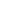Type Default Read Only Description [C#] `UnitType` [Visual Basic] `UnitType` UnitType.Points No The current measurement units.Notes

This property holds the current measurement units.

The UnitType enumeration may take the following values:

• Points (PostScript Points) - 1/72 of an Inch
• Twips (Twentieths of a Point) - 1/20 of a PostScript Point
• Didots (Didot Points) - 1/72 of a French Royal Inch
• ATAPoints (ATA Points) - 1/72.272 of an Inch
• TeXPoints (TeX Points) - 1/72.27 of an Inch
• INPoints (l'Imprimerie Nationale Points) - 0.4 mm
• Picas - 12 PostScript Points
• Ciceros - 12 Didot Points
• ATACiceros - 12 ATA Points
• TeXCiceros - 12 TeX Points
• Microns - millionths of a metre
• Mm - thousandths of a metre
• Cm - hundredths of a metre
• M - metres
• Inches
• Feet

There are a variety of methods you can use to change coordinate systems.

 Why are my Units a string? In older versions of ABCpdf the Units property was a string. So you might find code of this form.   ```theDoc.Units = "mm"; ``` ```theDoc.Units = "mm" ``` In Version 8 the Units property has been changed to a true enumeration. This is a safer way of coding as it allows the compiler to ensure that the values you are using are valid. Your new code should look like this.   ```theDoc.Units = UnitType.Mm; ``` ```theDoc.Units = UnitType.Mm ``` The names of the items in the UnitType enumeration are the same as the values of the strings used in previous versions. So changing your code should be a simple search and replace operation. Alternatively if you need to convert between enumerations and strings automatically you can do so. To convert from a string to an enumeration use the following code.   ```UnitType unitType = (UnitType)Enum.Parse(typeof(UnitType), unitString, true); ``` ```Dim unitType As UnitType = CType([Enum].Parse(GetType(UnitType), unitString, True), UnitType) ``` To convert from an enumeration to a string use the following code.   ```string unitString = unitType.ToString("G"); ``` ```Dim unitString As String = unitType.ToString("G") ```Example

 See the TopDown property.